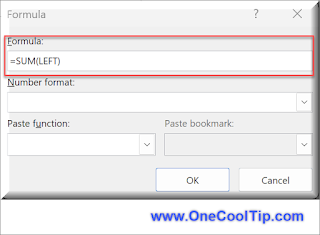## Monday, September 11, 2023

### How to Master Formulas in Microsoft Word

Have you ever wondered how to make your tables in Word more dynamic and functional?

Here's a Cool Tip:  Use the Formula feature in Microsoft Word.

That's right.

Formulas in Microsoft Word.

Microsoft Word offers a powerful feature that allows you to use formulas within tables, much like in Excel.

Let's walk through  the process of using the formula function in Microsoft Word and explain the concept of positional arguments.

Here's how to do it.
• Insert a table into your Word document where you want to perform calculations.
• Fill the cells with the data you want to calculate.
• Click on the cell where you want the result of your calculation to appear.
• If the cell is not empty, delete its contents.
• Go to the Layout tab, in the Data group, and click on Formula.
• Begin your formula with an equal sign, followed by your chosen function.fig. 2 - Word Formula
• Enter a function in the Formula field, such as SUM, AVERAGE, COUNT, MAX, MIN, PRODUCT.
• Click on Paste function to select a function.
• Use Positional Arguments: In the parentheses that follow the function, add positional arguments like LEFT, RIGHT, ABOVE, BELOW
• These refer to the relative position of the cells that you want to include in your calculation.
Notes:

• Update Formula Results
• The result of a formula is calculated when it is inserted and when the document containing the formula opens. You can also manually update the result of one or more specific formulas by selecting them and pressing F9.
• Understanding Positional Arguments
• Positional arguments are used to refer to cell positions relative to the cell containing the formula. For example, if you want to sum numbers in a column above a specific cell, you would use the argument ABOVE in your SUM function.
With these steps, you can now perform calculations right within your Word document.

No more switching back and forth between Word and Excel for simple calculations!

Enjoy!